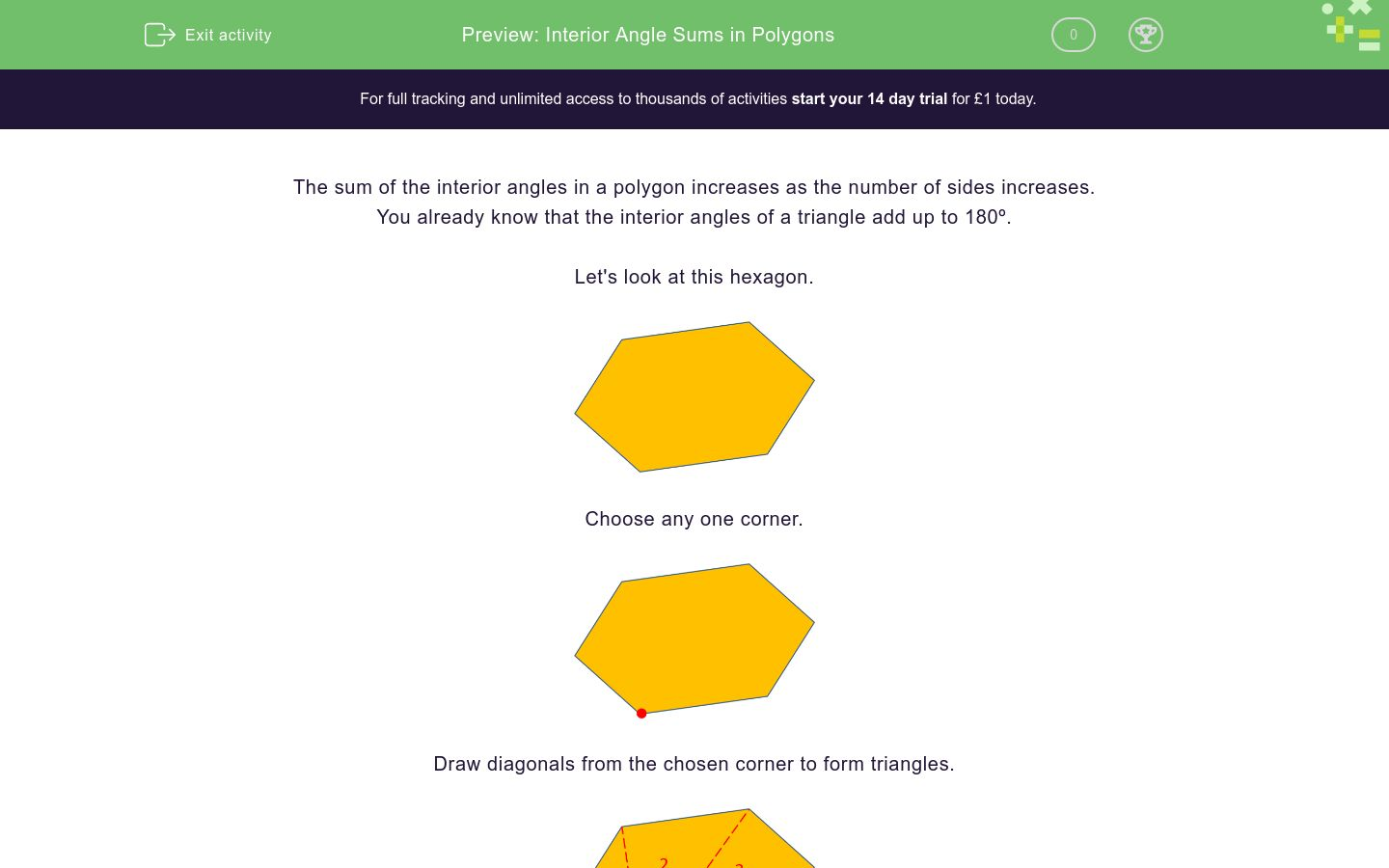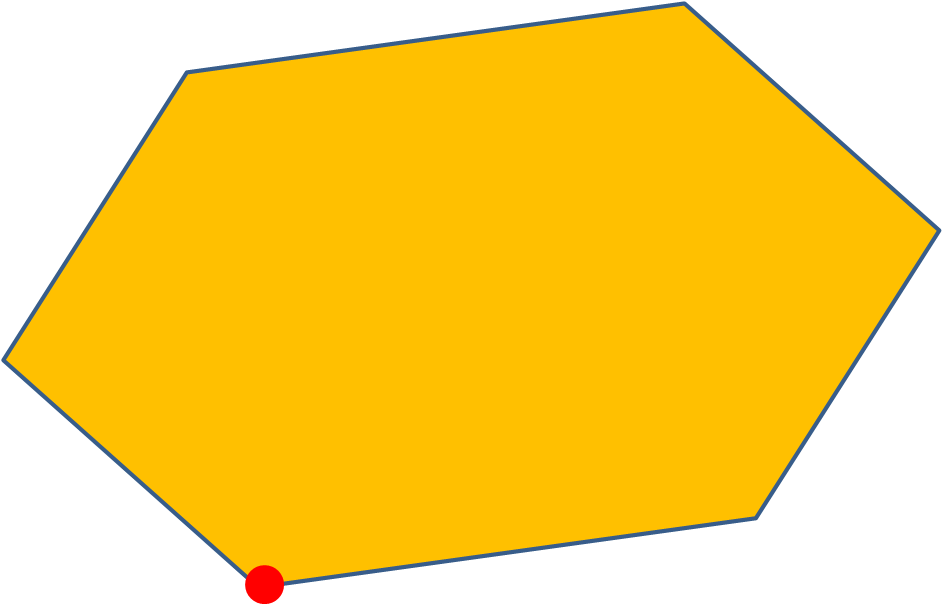# Interior Angle Sums in Polygons

In this worksheet, students apply their knowledge of triangles to calculate the number of sides in a polygon given the sum of its interior angles.Key stage:  KS 4

Curriculum topic:  Geometry and Measures

Difficulty level:### QUESTION 1 of 10

The sum of the interior angles in a polygon increases as the number of sides increases.

You already know that the interior angles of a triangle add up to 180º.

Let's look at this hexagon.Choose any one corner.Draw diagonals from the chosen corner to form triangles.There are 4 triangles inside the hexagon.

4 x 180º = 720º

The sum of the interior angles of a hexagon is 720º.

No. of triangles = No. of sides - 2

Example

Find the number of sides in a polygon whose interior angle sum is 720º.

720 ÷ 180 = 4

No. of sides = No. of triangles + 2

No. of sides = 4 + 2 = 6

Find the number of sides in a polygon whose interior angle sum is 180º.

Find the number of sides in a polygon whose interior angle sum is 540º.

Find the number of sides in a polygon whose interior angle sum is 360º.

Find the number of sides in a polygon whose interior angle sum is 1080º.

Find the number of sides in a polygon whose interior angle sum is 1440º.

Find the number of sides in a polygon whose interior angle sum is 900º.

Find the number of sides in a polygon whose interior angle sum is 1800º.

Find the number of sides in a polygon whose interior angle sum is 2340º.

Find the number of sides in a polygon whose interior angle sum is 4500º.

Find the number of sides in a polygon whose interior angle sum is 10440º.

• Question 1

Find the number of sides in a polygon whose interior angle sum is 180º.

3
EDDIE SAYS
180 ÷ 180 + 2
• Question 2

Find the number of sides in a polygon whose interior angle sum is 540º.

5
EDDIE SAYS
540 ÷ 180 + 2
• Question 3

Find the number of sides in a polygon whose interior angle sum is 360º.

4
EDDIE SAYS
360 ÷ 180 + 2
• Question 4

Find the number of sides in a polygon whose interior angle sum is 1080º.

8
EDDIE SAYS
1080 ÷ 180 + 2
• Question 5

Find the number of sides in a polygon whose interior angle sum is 1440º.

10
EDDIE SAYS
1440 ÷ 180 + 2
• Question 6

Find the number of sides in a polygon whose interior angle sum is 900º.

7
EDDIE SAYS
900 ÷ 180 + 2
• Question 7

Find the number of sides in a polygon whose interior angle sum is 1800º.

12
EDDIE SAYS
1800 ÷ 180 + 2
• Question 8

Find the number of sides in a polygon whose interior angle sum is 2340º.

15
EDDIE SAYS
2340 ÷ 180 + 2
• Question 9

Find the number of sides in a polygon whose interior angle sum is 4500º.

27
EDDIE SAYS
4500 ÷ 180 + 2
• Question 10

Find the number of sides in a polygon whose interior angle sum is 10440º.

60
EDDIE SAYS
10440 ÷ 180 + 2
---- OR ----

Sign up for a £1 trial so you can track and measure your child's progress on this activity.

### What is EdPlace?

We're your National Curriculum aligned online education content provider helping each child succeed in English, maths and science from year 1 to GCSE. With an EdPlace account you’ll be able to track and measure progress, helping each child achieve their best. We build confidence and attainment by personalising each child’s learning at a level that suits them.

Start your £1 trial